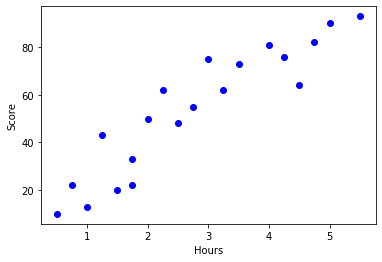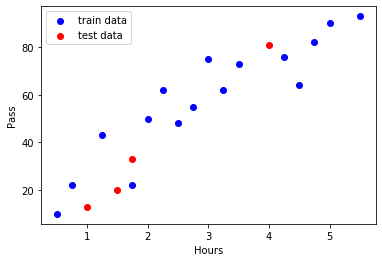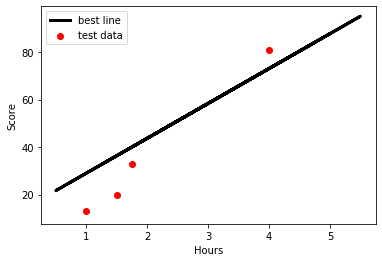## python实现线性回归（一）_python画图x.y轴的0画成一个_Xindolia_Ring的博客-程序员宅基地

``````import pandas as pd
import numpy as np
import matplotlib.pyplot as plt
from pandas import DataFrame,Series
from collections import OrderedDict
from sklearn.model_selection import train_test_split
from sklearn.linear_model import LinearRegression

#创建数据集
examDict  = {'学习时间':[0.50,0.75,1.00,1.25,1.50,1.75,1.75,2.00,2.25,2.50,2.75,3.00,3.25,3.50,4.00,4.25,4.50,4.75,5.00,5.50],
'分数':[10,22,13,43,20,22,33,50,62,48,55,75,62,73,81,76,64,82,90,93]}

#将上面的字典变为有序字典
examOrderedDict=OrderedDict(examDict)
#将有序字典读取为pandas中的二维数组
examDf=pd.DataFrame(examOrderedDict)

#提取二维数组中的特征值作为x轴
exam_X=examDf.loc[:,'学习时间']
#提取二维数组中的特征值作为y轴
exam_y=examDf.loc[:,'分数']

#绘制散点图
plt.scatter(exam_X,exam_y, color='b', label='Exam score')
#plt.scatter(examDf.分数,examDf.学习时间,color = 'b',label = "Exam Data")

#添加图的标签（x轴，y轴）
plt.xlabel("Hours")
plt.ylabel("Score")
#显示图像
plt.show()````````````#使用corr()计算相关系数，返回值为一个相关系数矩阵
rDf = examDf.corr()
print(rDf)``````
``` 学习时间        分数

```
``````#将原数据集拆分训练集和测试集
X_train,X_test,Y_train,Y_test = train_test_split(exam_X,exam_y,train_size=0.8)
#X_train为训练数据标签,X_test为测试数据标签,exam_X为样本特征,exam_y为样本标签，train_size 训练数据占比

print("原始数据特征:",exam_X.shape,",训练数据特征:",X_train.shape,",测试数据特征:",X_test.shape)
print("原始数据标签:",exam_y.shape, ",训练数据标签:",Y_train.shape,",测试数据标签:",Y_test.shape)

#散点图
plt.scatter(X_train, Y_train, color="blue", label="train data")
plt.scatter(X_test, Y_test, color="red", label="test data")

#添加图标标签
plt.legend(loc=2)
plt.xlabel("Hours")
plt.ylabel("Pass")
#显示图像
plt.show()``````
```原始数据特征: (20,) ,训练数据特征: (16,) ,测试数据特征: (4,)

`````````model = LinearRegression()

#对于模型错误我们需要把我们的训练集进行reshape操作来达到函数所需要的要求
# model.fit(X_train,Y_train)

#reshape如果行数=-1的话可以使我们的数组所改的列数自动按照数组的大小形成新的数组
#因为model需要二维的数组来进行拟合但是这里只有一个特征所以需要reshape来转换为二维数组
X_train = X_train.values.reshape(-1,1)
X_test = X_test.values.reshape(-1,1)

#训练模型
model.fit(X_train,Y_train)

#截距
a  = model.intercept_
#回归系数
b = model.coef_

print("最佳拟合线:截距",a,",回归系数：",b)``````
`最佳拟合线:截距 14.421286031042136 ,回归系数： [14.65809313]`
``````#训练数据的预测值
y_train_pred = model.predict(X_train)
#绘制最佳拟合线：标签用的是训练数据的预测值y_train_pred
plt.plot(X_train, y_train_pred, color='black', linewidth=3, label="best line")

#测试数据散点图
plt.scatter(X_test, Y_test, color='red', label="test data")

#添加图标标签
plt.legend(loc=2)
plt.xlabel("Hours")
plt.ylabel("Score")

#显示图像
plt.show()

score = model.score(X_test,Y_test)
print('score:',score)```````score: 0.7724491634122271`

### Ros2 TF工具_tf2_ros::buffer_冻★结♬ ☞memory☜的博客-程序员宅基地

ROS2 TF工具使用_tf2_ros::buffer

### [编译原理]LL(1)分析法+例题 学习_踩踩芽的博客-程序员宅基地

LL(1)分析法LL(1) 分析法又称预测分析法 ，是 一种不带回溯的非递归自上而下分析法。LL(1)分析器LL(1)分析表LL(1)文法：分析表M不含多重定义入口的文法1、一个LL(1)文法所定义得语言恰好就是它的分析表所能识别的全部句子。2、一个上下文无关文法是LL(1)文法的充要条件（判断一个文法是否是LL(1)文法）：对每一个非终结符A的任何两个不同的产生式A→α|β，有下面条件（都是避免了多重入口）成立（1）FIRST(α) ∩ FIRST(β) = ∅：A的每个候选是都不存_ll(1)分析法

### 第九章： TypeScript-for of循环_typescript for of_小仙女de成长的博客-程序员宅基地

forEach()for infor offorEach 这边只能打印出来1234 desc不能打出来var myArray = [1,2,3,4];myArray.desc = " four number ";myArray .forEach( value =&gt; console.log(value));for in 这个打印出来的是数组的下标跟属性的名字,也可以打印出..._typescript for of

### JAVA学习，常用常记（基本数据类型补充（包装类，min，max），转义，三种变量类型，静态成员函数和类变量（菜鸟教程补充））_在java中定义公有的int类型常量min是什么_hhjian6666的博客-程序员宅基地

Java 的两大数据类型:内置数据类型 引用数据类型public class PrimitiveTypeTest { public static void main(String[] args) { // byte System.out.println("基本类型：byte 二进制位数：" + Byte.SIZE); ..._在java中定义公有的int类型常量min是什么